### Home > CALC > Chapter 6 > Lesson 6.3.2 > Problem6-91

6-91.
1. Examine the integrals below. Consider the multiple tools available for evaluating integrals and use the best strategy for each. After evaluating the integral, write a short description of your method. Homework Help ✎

1.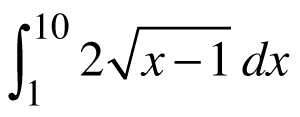2.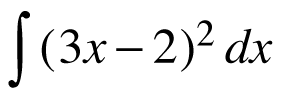3.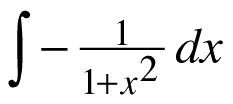4.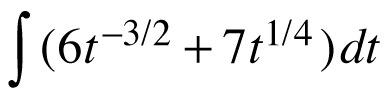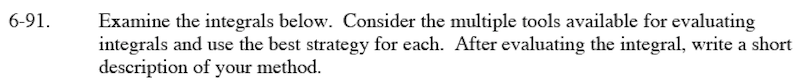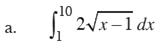Convert the radical to an exponent. Then use the form upowerdu.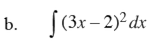If u = (3x − 2), then you need to add a du. In this case, it would be 3 dx.
Since you already have a dx, add a 3 taking out a (1/3) to compensate.

Multiply (3x − 2) (3x − 2).
Then take the antiderivative.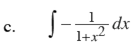Use the form for one of the inverse trig. functions.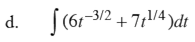Take the antiderivative.